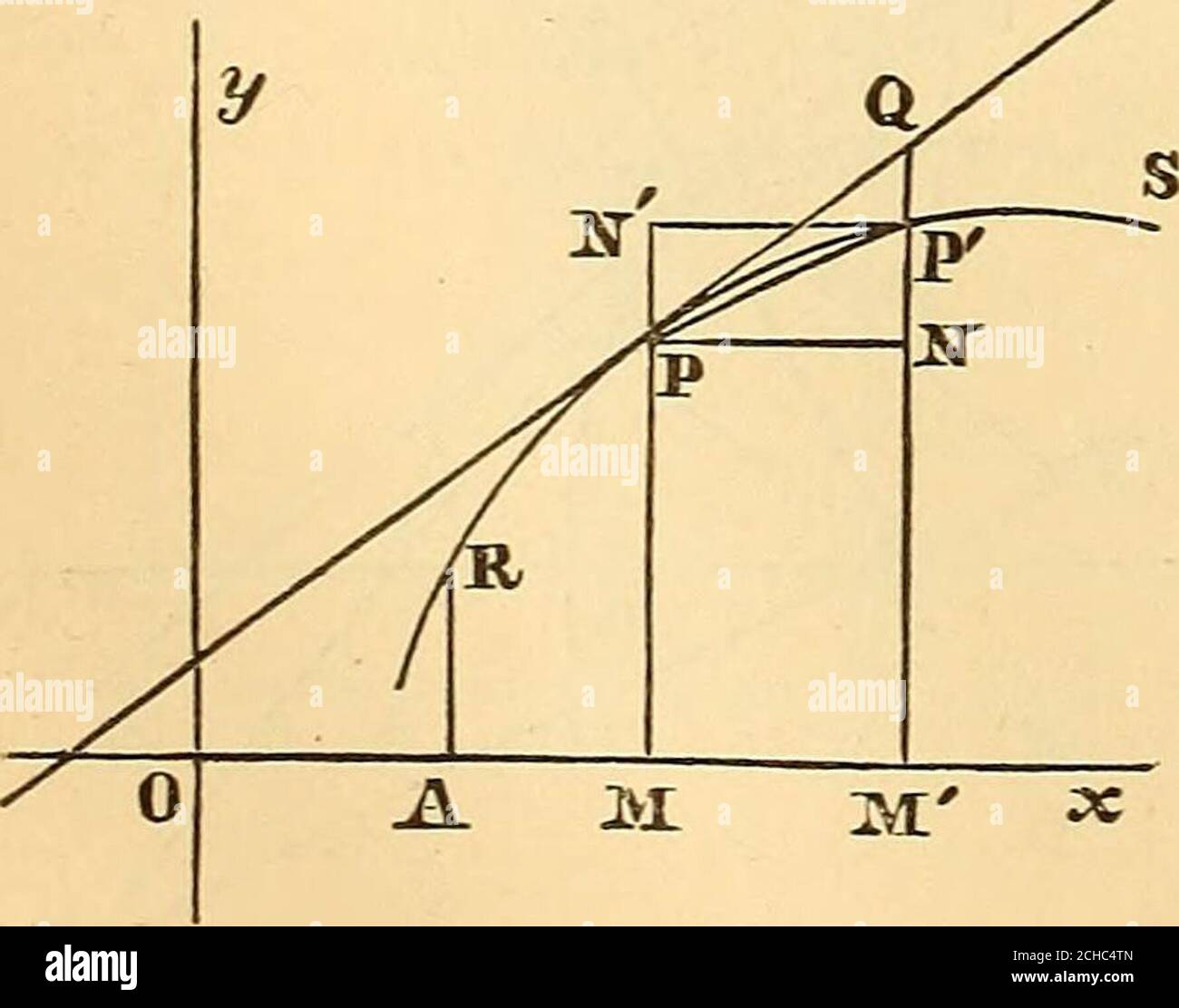# . A new treatise on the elements of the differential and integral calculus . re, and the radii vectoresF3I^= r, FS^=^ r^ as radii, describe the arcs MIy SK, limitedby these radii vectores. We have sector PJ/7 &lt; a?^ SX; or, since sector PJ/J =7^ r-A^, and sector P/S^ r^^^, --r-A^&lt; ^u &lt;-r^2^6; 1 &lt; — &lt; T ^ A^ ^ 2 But, at the limit, r becomes equal to r: hence du 1 , du 1= -r^dd. y The equations ic=rrcos./9, ?/ = rsin. (9, give - = tan. (9; whence,by differentiating with respect to 6^ dy dx ^ddydo cos.-^(? r^ COS.!cos.-(? = 7-, ^(^xdy — ijdx) zzz-r^dd; an expression in terms of rec## Image details

Contributor:

Reading Room 2020 / Alamy Stock Photo

Image ID:

2CHC4TN

File size:

Releases:

Model - no | Property - noDo I need a release?

Dimensions:

1783 x 1402 px | 30.2 x 23.7 cm | 11.9 x 9.3 inches | 150dpi

This image is a public domain image, which means either that copyright has expired in the image or the copyright holder has waived their copyright. Alamy charges you a fee for access to the high resolution copy of the image.

This image could have imperfections as it’s either historical or reportage.

. A new treatise on the elements of the differential and integral calculus . re, and the radii vectoresF3I^= r, FS^=^ r^ as radii, describe the arcs MIy SK, limitedby these radii vectores. We have sector PJ/7 &lt; a?^ SX; or, since sector PJ/J =7^ r-A^, and sector P/S^ r^^^, --r-A^&lt; ^u &lt;-r^2^6; 1 &lt; — &lt; T ^ A^ ^ 2 But, at the limit, r becomes equal to r: hence du 1 , du 1= -r^dd. y The equations ic=rrcos./9, ?/ = rsin. (9, give - = tan. (9; whence, by differentiating with respect to 6^ dy dx ^dd~ydo cos.-^(? r^ COS.!cos.-(? = 7-, ^(^xdy — ijdx) zzz-r^dd; an expression in terms of rectangular co-ordinates for the dif-ferential of a polar area that is of frequent use. IGG, Differential co-efficient of the volume of a solid ofrevolution. If V represent the volumegenerated by the revolution ofthe plane area ABPM about theaxis Ox, and x be increased by3I3I z=i ^x, the corresponding in-crement AFof Fwill be the vol-ume generated in the revolutionby the area MFFW. Now, ifA.r be so small, that y increases constantly from P to P, A V will. DIFFERENTIAL CO-EFFICIENTS OF VOLUMES. 279 be included between the volumes of the cylinders generated by the rectangles MPNM, MNFM. Hence, denoting MP by y, and MP by 7/1, we have Tiy^^x &lt; aF&lt; Tty^ acc, or ny^ &lt; -— &lt;^ Tty^] aVthat is^ — is comprised between the two quantities Tty^, tt?/^, the second of which converges to equality with the first as AX diminishes. Hence, at the limit, dV -^-zzzTty^, dV^^ny^dx. (XX 107, Differential co-efficient of the surface of a solid ofrevolution. Let s represent the arc PP (figure of last article), and Sthe surface generated by the revolution of this arc about theaxis Ox. For the increment M3P ^=Axofx, s will be increasedby the arc PP^ = A5; and S, by aS:=^ the surface generatedby AS. When AX is sufficiently small, the surface A^S^will becomprised between the surface of the conical frustum gener-ated by the chord PP^, and the surface generated by the brokenline PQP. The surfa

Save up to 70% with our image packs